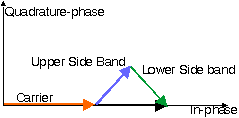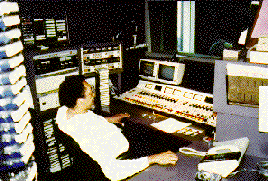### JPL's Wireless Communication Reference Website

#### Chapter: Analog and Digital Transmission# Analog Transmission over Fading Channels

## Amplitude modulation

Various methods exist to transmit a baseband message m(t) using an RF carrier signal c(t) = Ac cos ( omegac t + theta ). In linear modulation, such as Amplitude Modulation (AM) and Single Side band (SSB) the amplitude Ac is made a linear function of the message m(t). For AM, that is

s(t) = Ac (1+ c m(t)) cos ( ωc t )

where c is the modukation index (0 ≤ c ≤ 1) For instance, for full (c=1) tone modulation with

m(t) = cos ( ωm t),

we get

s(t) = Ac (1+ cos ( ωm t)) cos ( ωc t )

s(t) = Ac cos ( ωc t) + Ac/2 cos ( (ω c m)t ) + Ac/2 cos ( (ω c m)t )Figure: Phasor Diagram for AM with tonal modulation.

AM has the advantage that the detector circuit can be very simple. This allows inexpensive production of mediu wave broadcast receivers. The transmit power amplifier, however, needs to be highly linear and therefor expensive and power consuming.Photo: Control room and presenters booth of KGO newstalk 81, San Francisco's most popular radio station. Because of its limited audio quality, AM broadcast radio is mostly used for news and information formats.

For mobile reception of AM audio signals above 100 MHz, the spectrum of channel fluctuations due to fading and in the message overlap. Hence the Automatic Gain Control in the receiver IF stages can not distinguish the message and channel fading. AGC will thus distort the message m(t).

AM is only rarely used for mobile communication, although it is still used for radio broadcasting. AM broadcasting uses the medium wave band 540-1600 kHz. Alse long wave and short wave broadcast bands are used.

## Single Side Band

In the frequency power spectrum of AM signals we recognize an upper side band and a lower side sideband, with frequency components above and below the carrier at fc. In Single Side Band transmission, the carrier and one of these side bands are removed.

An SSB message can be recovered by multiplying the received signal by cos( omegac t + phi ). If the local oscillator has a phase offset (theta - phi), the detected signal is a linear combination of the original message m(t) and a 90 degree phase-shifted version (its Hilbert transform). The human ear is not very sensitive to such phase distortion; therefor the detected signal sounds almost identical to m(t), despite any phase offset. However, such phase shifts make SSB unsuitable for digital transmission.

The effect of a frequency error in the local oscillator is more dramatic to analog speech signals. Its effect can best be understood from the frequency domain description of the SSB signal. A frequency shift of all baseband tones occurs. In this case, the harmonic relation between audio tones is lost and the signal sounds very artificial.

SSB is relatively sensitive to interference, which requires large frequency reuse spacings and severely limits the spatial spectrum efficiency of cellular SSB networks.

AGC to reduce the effect of amplitude fades substantially affects the message signal. Furthermore, SSB requires very sharp filters, which are mostly sensitive to physical damage, temperature and humidity changes. This makes SSB not very attractive for mobile communication.

## Phase Modulation

In phase modulation, the transmit signal has the constant-amplitude form s(t) = Ac cos ( ωc t + constant m(t)) where constant is the called the phase deviation.

###Exercise

Show that for Narrowband Phase Modulation (NBPM) with constant << 1, Phase modulation can be approximated by the linear expression s(t) = Ac cos (ωc t) + m(t) cos (ωc t). Compare NBPM with AM
• by drawing the phasor diagrams,
• by computing the transmit power in the carrier and each sideband,
• by calculating the signal-to-noise ratio for coherent detection.
• Explain why in mobile communications NBPM has advantages over AM.

## Frequency Modulation

For frequency deviation delta_f , the transmit signal is of the form
```                                    t
x(t)=Ac cos(omegac t+ 2pi delta_f int  m(x)dx)
-inf
```

That is, FM can be implemented by a PM modulator, and an integrator for the message signal.See an animation of the phasor diagram for FM modulation.

For a message bandwidth W, the transmit bandwidth Bt can be approximated by the Carson bandwidth Bt = 2 ( deltaf + W)

In the event of 2W < deltaf < 10 W, a better approximation is Bt = 2 ( deltaf + 2 W)

###Exercise

Find Bt for FM broadcasting with deltaf = 75 kHz and W = 15 kHz. Cellular telephone nets with speech bandwidth W = 3000 Hz typically transmit over Bt = 12.5 or 25 kHz. Find the frequency deviation deltaf . Compare the use of 12.5 and 25 kHz bandwidth in terms of implementation difficulty, audio quality, vulnerability to interference, reuse factors needed and spectrum efficiency.

After frequency-nonselective multipath propagation, a received FM signal

• contains random phase and frequency modulation, which are additive to the modulation
• suffers from dispersion. The audio signal will be distorted.FM is used for broadcasting in the 87.5 to 108 MHz band. The modulation signal, as fed into the transmitter, consists of
• Monophonic (Left + Right) audio in baseband (50-15.000 Hz)
• A pilot tone at 19 kHz
• Stereophonic (Left - Right) audio DSB modulation (AM without carrier) at 38 kHz (= 2 x 19 kHz)
• Subcarrier data, typically at 57 kHz (= 3 x 19 kHz), e.g. RDS
All audio is prefiltered (pre-emphasis) to improve the signal-to-noise ratio. This filter boosts high frequencies above 2 or 3 kHz.

### Reception above the FM-Threshold

If the signal-to-noise ratio is sufficiently large, the received signal is dominated by the wanted signal. The effect of noise can be approximated as a linear additive disturbance. The minimum signal-to-noise ratio for which this assumption is reasonable is called the FM capture threshold.

### Capture

In non-linear modulation, such as phase modulation (PM) or frequency modulation (FM), the post-detection signal-to-noise ratio can be greatly enhanced as compared to baseband transmission or compared to linear modulation. This enhancement occurs as long as the received pre-detection signal-to-noise ratio is above the threshold. Below the threshold the signal-to-noise ratio deteriorates rapidly. This is often perceived if the signal-to-noise ratio (C/N) increases slowly: a sudden transition from poor to good reception occurs. The signal appears to "capture" the receiver at certain C/N. A typical threshold value is 10 (10 dB) C/N at RF. The audio SNR where capture occurs depends on the frequency deviation.

### Effects of Rayleigh fading on FM reception

In a rapidly fading channel, the events of crossing the FM capture threshold may occur too frequently to be distinguished as individual drop outs. The performance degradation is perceived as an average degradation of the channel. The capture effect and the FM threshold vanish in such situations.

### Effect of Amplitude variations

Fluctuations of the signal-to-noise ratio cause fluctuations of received noise power and fluctuations of the amplitude of the detected wanted signal. Some analyses assume that the difference between the detected signal and the expected signal is perceived as a noise type of disturbance. It is called the signal-suppression 'noise', even though disturbances that are highly correlated with the signal are mostly perceived as 'distortion' rather than as noise.

### Effect of Random FM

For large local-mean signal-to-noise ratios Random FM is the only remaining factor. For voice communication with audio passband 300 - 3000 Hz, the noise contribution due to random FM leads to a SNR of
```         2          Freq. deviation
SNR =  ---- Square (---------------) S
```
with S the audio power at the detector output. This does not depend on additive prediction noise. Wideband transmission (large frequency deviation) is thus significantly less sensitive to random FM than narrowband FM.

###Exercise:

A national regulatory agency meets to decide upon transmission standards for a cellular telephone network. Which parties should be consulted in this process? Compare different modulation techniques from the point of view of the network operator, the terminal manufacturing industry, the network manufacturing industry, the customer, the international regulatory agency allocating frequency bands, the computer industry interested in developing additional data transmission schemes.

###Exercise:

Review techniques for computing outage probability. Show that the optimum spectrum efficiency is achieved by minimizing z^beta Bt2 where z is the receiver capture threshold and beta is the path loss law. Optimize the FM frequency deviation for a given beta . Compare the spectrum efficiency of the Swedish NMT 450 MHz cellular network (using freq. dev. of 25 kHz) with the Dutch system using a deviation of 12.5 kHz. Is it true that the Dutch can accommodate twice as many subscribers in the same piece of spectrum?

###Exercise:

Summarize the advantages of digital transmission over analog.